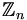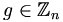# ComputerNetworkingandPracticalSecurity

Fall 2006

## Contents:

• Zero-Knowledge Proofs
• Theory
• Example Using Basic Graph Theory
• RSA
• Basic Group Theory and Definitions
• The Algorithm
• Why it Works

## Zero-Knowledge Proofs

### Theory

Zero-knowledge proofs are generally used for authentication. Similar to public/private key encryption, person A ("Alice") has a secret, and she wants to convince person B ("Bob") that she knows it without actually revealing the secret or any information about it. The methods for doing this are not standard, but they revolve around some probabilistic argument. For any probability p (less than 1), Bob must be able to verify Alice's claim to that degree of certainty (conversely Alice cannot be able to fake the secret). Usually this is done through rounds, where Bob is allowed to ask some question at random relating to the secret.

## ExampleFirst, some quick graph theory definitions. A graph is a mathematical structure comprised of vertices and edges. Visually we represent this as points (vertices) connected by lines (edges), although we can also represent it as a table (which is usually easier when passing data back and forth on computers). If a graph has a route of edges traversing it, passing through each vertex exactly once, we call this route a Hamiltonian path. A graph can have many Hamiltonian paths, or none at all, and generally they are difficult to find.
A mapping, or isomorphism, of a graph G is an operation that jumbles the vertices of G while preserving the edge relations -- i.e. if vertices A and B have a connecting edge and get mapped to E and F, E and F are still connected. The result is a second graph H, which visually won't necessarily resemble G, but is isomorphic to G -- i.e. can be reverse-mapped back to G.
So say we have a graph G which is public knowledge. Alice's secret is a Hamiltonian path in G (she probably generated G herself around the Hamiltonian path rather than searched for a path in G). At the start of each round, Alice creates a mapping "G->H" and gives H to Bob (she keeps f(G) secret).Bob then gets to ask one of two questions, which he picks at random: either he asks for f(G), or he asks for a Hamiltonian path in H. Since the structure of G is preserved in H, Alice can map her path in G to a corresponding on in H. If Bob asks for f(G), he can follow it backwards to verify H is actually isomorphic to G. If he asks for the Hamiltonian path, he simply verifies that the path is valid in H. Alice and Bob repeat this process with a new mapping each time until Bob is satisfied.
Since Alice doesn't know which question Bob is going to ask, it is nearly impossible to give Bob a false positive. Say Alice is trying to trick Bob. Then for each round she can either create a valid mapping f(G), or she can create a new graph that isn't isomorphic to G but which she does know a Hamiltonian path for. If Bob asks the right question, Alice can give Bob an answer that he can verify, but since Bob's choice is random, she only has a 1/2 chance per round of fooling Bob. So for n rounds, the chance that Alice can give a false positive is (1/2)^n. For 3 rounds, Alice has a 1/8 chance of fooling Bob. For 10 rounds, the chance is only 1/1024. Of course if Alice can make some predictions about which question Bob will ask, her chances get better. Unless Bob is actually flipping a coin for each round, then he's probably using some computer based pseudo-random number generator. For a painful reminder on the actual randomness of pseudo-random number generators, see .

## RSA

### Basic Group Theory and Definitions

A group is a set that has an operation and follows certain properties. The operation is usually called either addition or multiplication, although the operation may differ greatly from the arithmetic one we're used to (for example, compare with matrix addition and multiplication). For the rest of this section we will call the operation addition, since we will need to use multiplication as well soon.
The properties a simple group must adhere to are:
• Closure. For any a,bG , then (a + b)∈G .
• Associativity. (a + b) + c = a + (b + c) .
• Additive Identity. There exists some element "0" such that a + 0 = 0 + a = a .
• Additive Inverse. For every a , there exists some a − 1 such that a + a − 1 = 0 .
In addition to this, a group can also have a commutative property: a + b = b + a . If this property holds, then the group is called Abelian. Clearly most of these properties are the same as those you learned in basic algebra, which makes sense, since the real numbers under addition are in fact an Abelian group!
A ring is a group with an additional set of properties defined for a second operation (multiplication). Multiplicative closure, associativity, commutativity, and identity are defined the same as their addition counterparts (with the identity being defined as "1"). In addition to these, there is a distributive property stating that (a + b) * c = a * c + b * c .
An important example of a ring is, or the integers modulo some n . The modulo operator should be a familiar programming concept as the remainder of division by n , but to more formally define it, think of the elements of, the integers from 0 to n − 1 , being placed in order around a clock. If we think of our group in this circular pattern, the result of addition becomes clear without having to resort to division which we haven't formally defined (of course when performing modulo arithmetic in your head, it's often easier to just divide at the end).
Before we start applyingto the RSA algorithm, we need two more definitions. First, two numbers are relatively prime, or coprime, if they have no common divisor greater than 1. Second, in, the "totient function" φ(n) is defined as the number of elementswhere g and n are coprime. An important result of this definition is that if n is prime, φ(n) = n − 1 . You should also be able to take on faith that if n is the product of two primes p and q , then φ(n) = (p − 1)(q − 1) . Furthermore, aφ(n) = 1(modφ) . For proofs of the above results, as well as more information on the totient fuction, see .

### The Algorithm

The actual RSA algorithm is actually quite simple (though it relies on more theory than we have just covered). Unless otherwise noted, all operations are done in. Pick n as the product of two primes, p and q . Then find an a and b such that a * b = 1(modφ(n)) . Let (n,b) be your public key, and (p,q,a) be your private key. Then to encrypt your data, take its numerical encoding x (it doesn't matter how you encode it as long as you're consistent) and raise it to the a-th power. To decrypt it, the receiver raises the encrypted data to the b-th power ((xa)b ). This method provides authentication. Conversely if you're sending secret data to the owner of this key, you can encrypt with b and only they can decrypt with a .

### Why It Works

Raising a value to the a and then b is the same as (xa)b = xa * b . By our choice for a and b , we can rewrite this again as xφ(n) * t + 1 for some t . Then math>x^{\phi(n)*t + 1} = (x^{\phi(n)})^{t} * x = 1^{t} * x = 1 * x = x. So our encryption and decryption method works.
The security of the algorithm comes from the fact that factoring integers is hard, especially when there are only two factors. Without this information, an eavesdropper has no way of calculating φ(n) or a . For a more in depth look at this problem, as well as a handy algorithm for finding a and b , see .